1. Probability Distributions
2. Interpretations
3. Supply Chain Optimization
4. Probabilistic Programming
``````dice = uniform [1..6]
``````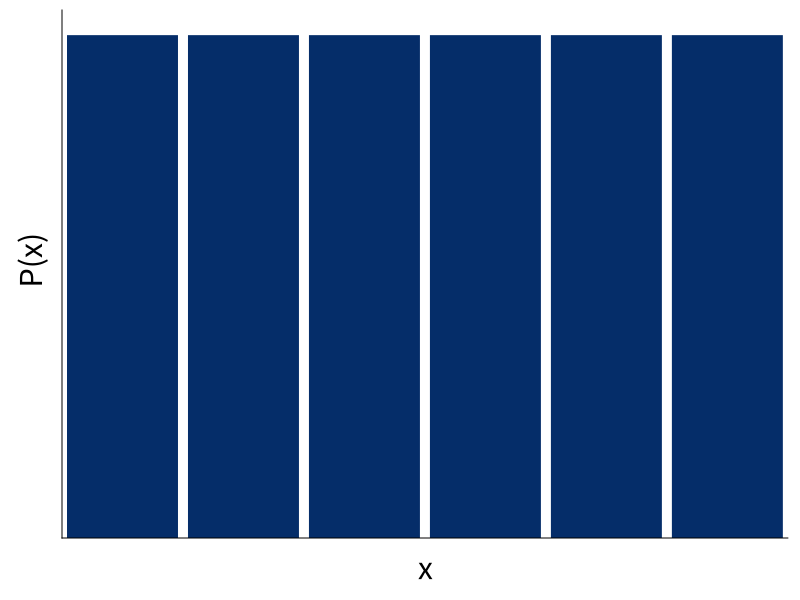``````dice2 = dice + dice
``````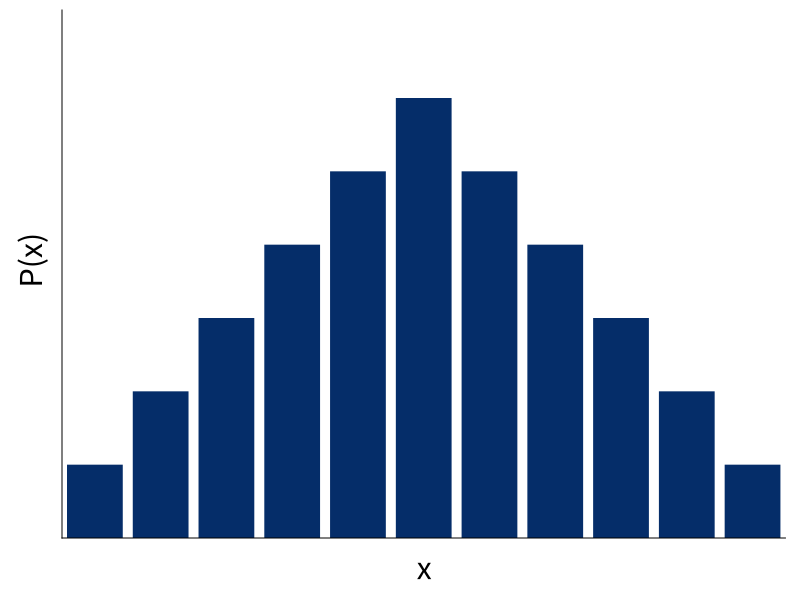## Abstract Type

``````data Dist a = ...

dice ∷ Dist Int
dice = uniform [1..6]

coin ∷ Double → Dist Coin
coin p =
weighted [(T, p), (H, 1 - p)]
``````

``````pure ∷ a → Dist a
fmap ∷ (a → b) → (Dist a → Dist b)
join ∷ Dist (Dist a) → Dist a
``````
• remember `flatMap` or `bind`:
``````x >>= f = join (fmap f x)
``````

## pure

``````pure x = uniform [x]
``````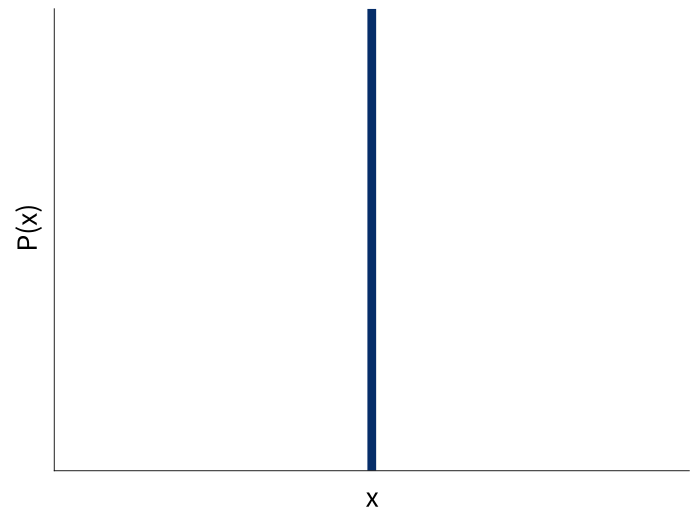## fmap

``````fmap (`mod` 5) dice2
``````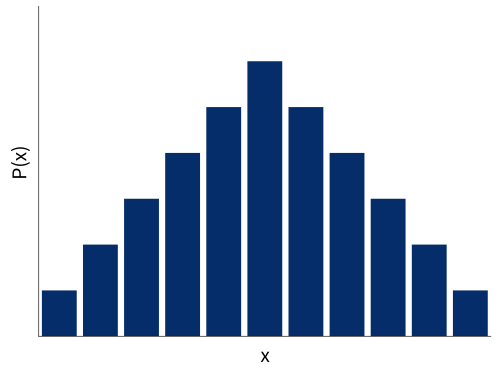## join

``````join ∷ Dist (Dist a) → Dist a
``````

Two different interpretations.

``````data Coin = H | T

coin ∷ Double → Dist Coin
coin p = weighted [(T, p), (H, 1 - p)]

fair   = coin 0.5
unfair = coin 0.9
``````
``````randomCoin ∷ Dist (Dist Coin)
randomCoin =
weighted [ (fair,   0.5)
, (unfair, 0.5)
]
``````
``````join randomCoin ∷ Dist Coin
``````

## Sampling

``````randomCoin ∷ Dist (Dist Coin)
randomCoin = …
``````
``````flattened ∷ Dist Coin
flattened = do
coinDist ← randomCoin
result   ← coinDist
return result
``````

## Exhaustive

``````fair, unfair ∷ Dist Coin
fair   = coin 0.5
unfair = coin 0.9
````````````randomCoin ∷ Dist (Dist Coin)
randomCoin = weighted [ (0.5, coin 0.5)
, (0.5, coin 0.9)
]
``````## flattened``````[ (H, 0.25), (T, 0.25)
, (H, 0.05), (T, 0.45) ]
``````
``````result = join randomCoin
``````## Sampling

pseudorandom number generators

``````sample ∷ Gen → (Double, Gen)

type Random a = State Gen a

run   ∷ Seed → Random a → a
runIO ∷ Random a → IO a
``````

## Exhaustive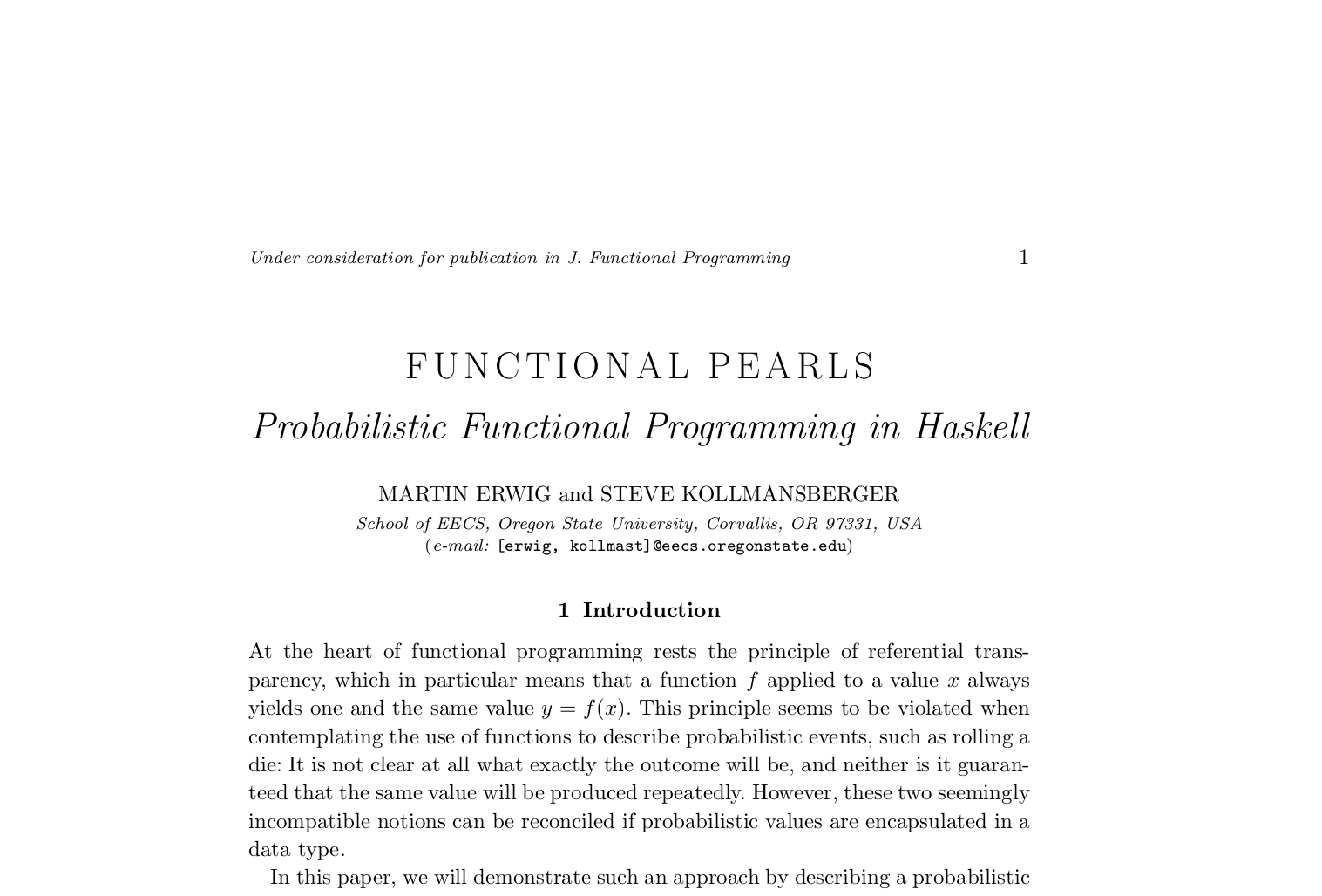## Exhaustive

``````type Probability = Double
-- or Rational or...

newtype Dist a = Dist
{ probabilities ∷ [(a, Probability)] }
``````

## Exhaustive

``````weighted ∷ [(a, Probability)] → Dist a
weighted = Dist

uniform ∷ [a] → Dist a
uniform xs = Dist (zip xs [1..])
``````

``````pure ∷ a → Dist a
pure x = Dist [(x, 1)]

join ∷ Dist (Dist a) → Dist a
join dists = Dist
[ (x, p₁ * p₂) | (d, p₁) ← dists
, (x, p₂) ← d ]
``````## Unnormalized## Normalizing

``````normalize ∷ Ord a ⇒ Dist a → Dist a
normalize = ...
``````

## Normalize automatically?

``````fmap (+) dice ∷ Dist (a → b)
``````
``````(+) <\$> dice <*> dice

((+) <\$> dice) ∷ Dist (a → b)
``````

• expressive
• intuitive

## Downsides

• sloooooow
• normalization

## Supply Chain Optimization## What is Target?

• 1806 stores
• 37 distribution centers
• Target.com

## Distribution Centers## Demand Uncertainty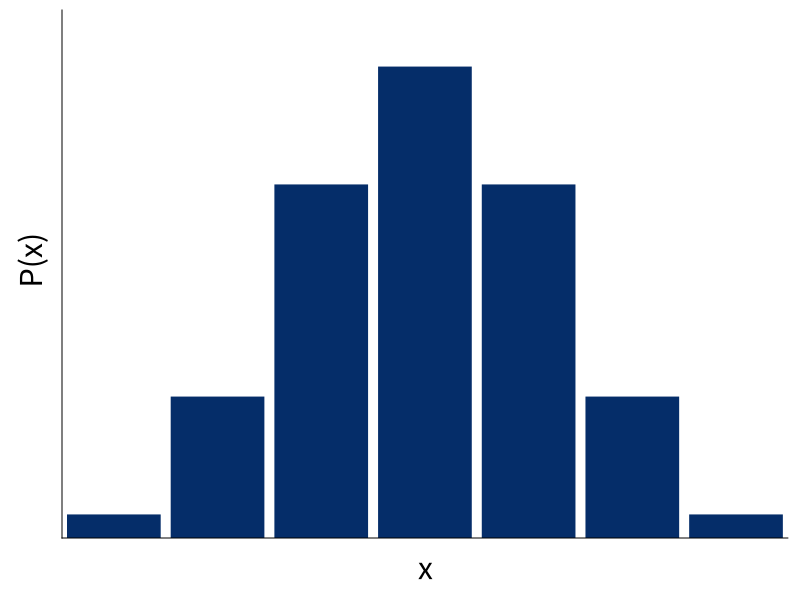## Demand Uncertainty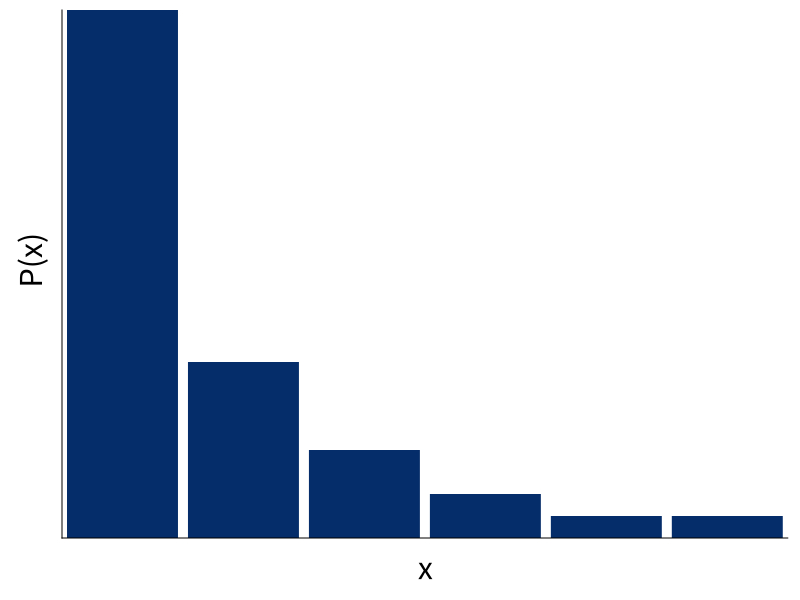``````class Monad m ⇒ MonadDist m where
weighted ∷ [(a, Probability)] → m a

uniform  ∷ [a] → m a
binomial ∷ Double → Int → m Int
{- etc -}
``````
``````instance MonadDist Dist
…
``````

## Models

• sampling:
• simulation
• simulation-based optimization
• exhaustive:
• linear programming
• dynamic programming

## Random

easy(ish) to try different generators

``````newtype Random a = Random {
runRandom ∷ ∀ m. PrimMonad m
⇒ Gen (PrimState m) → m a
} deriving Functor
``````

## Markov Decision Processes

• S: set of states
• A: set of actions
• P(s, a, s'): transition probability
• R(s, s'): reward

## Policy

• result of optimization
• function S → A
• maximizes expected reward

``````data MDP m s a r =
MDP { step ∷ s → a → m (r, s) }

type Policy s a = s → a
``````

## Simulation

``````data Markov m s r =
Markov { step ∷ s → m (s, r) }

apply ∷ MDP m s a r
→ Policy s a
→ Markov m s r
``````

## Example

``````step ∷ Qty → Qty → m (Qty, Money)
step inv order = do
let stocked = inv + order
cost    = price * order

let after  = max (stocked - buyers) 0
profit = price * (inv - after)

return (remaining, profit - cost)
``````

## Optimization Techniques

• dynamic programming (policy iteration)
• linear programming
• reinforcement learning
• features
• neural nets
• domain-specific algorithms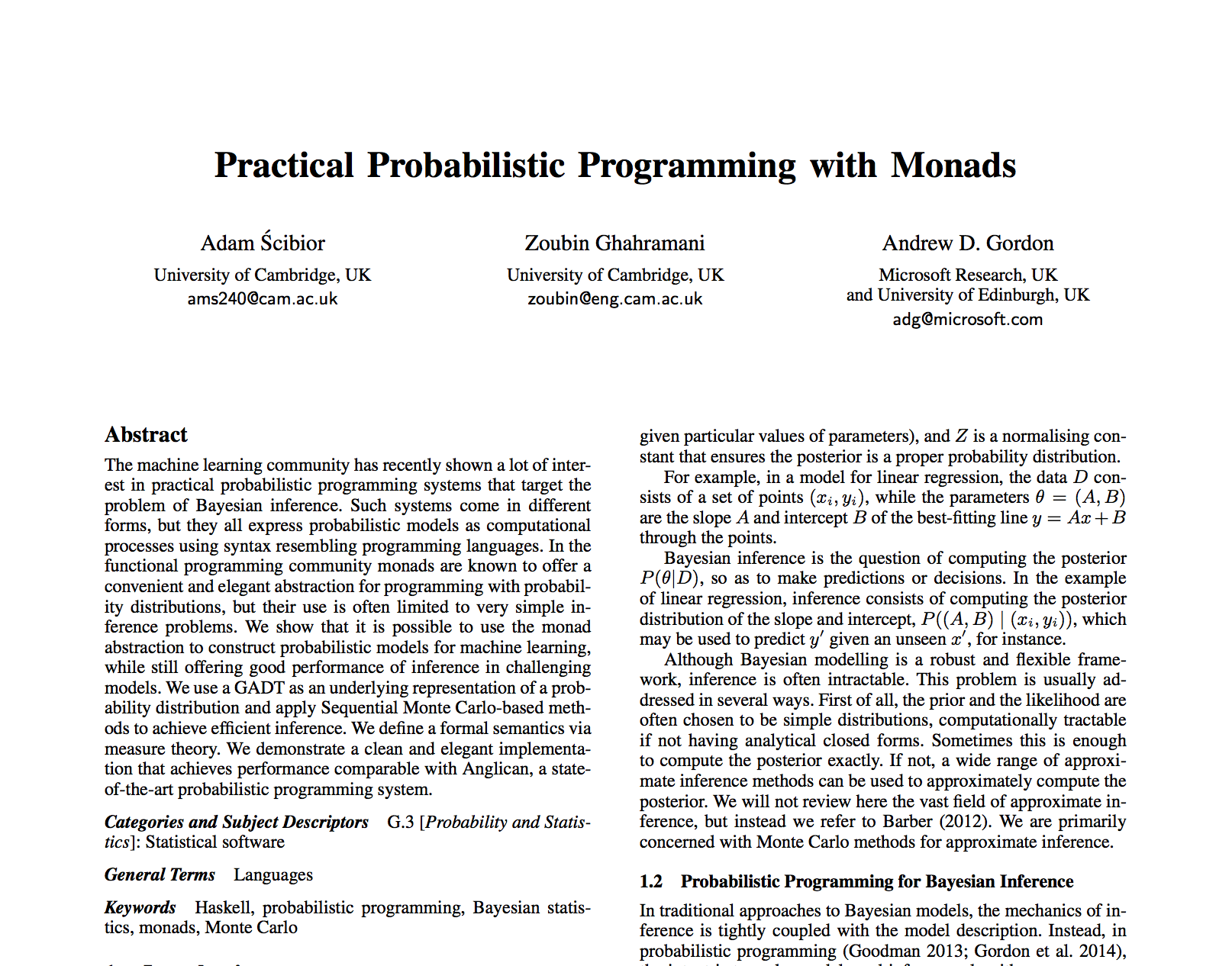``````data D a where
Return      ∷ a → D a
Bind        ∷ D b → (b → D a) → D a
Primitive   ∷ Sampleable d ⇒ d a → D a
Conditional ∷ (a → Prob) → D a → D a
``````
``````instance Monad Dist where
return = Pure
(>>=)  = Bind
``````

## Future?

• full-on probabilistic programming